# AP Calculus BC Practice Test 36

### Test Information10 questions20 minutes

Calculator Disallowed

1. What is the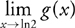, if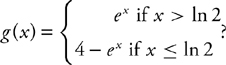2.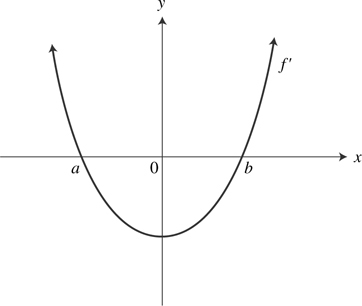The graph of f′ is shown above. A possible graph of f is (see below):

3. What is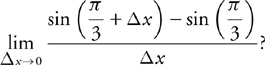?

4. If x = cos t and y = sin2t, then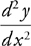at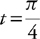is

5. If f (x) is an antiderivative of xe-x2 and f (0) = 1, then f (1) =

6.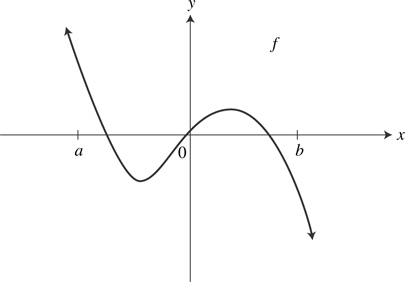The graph of the function f is shown above. Which of the following statements is/are true?

I. f′(0) = 0

II. f has an absolute maximum value on [a, b]

III. f″ < 0 on (0, b)

7.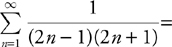8. Which of the following series are convergent?

I.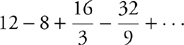II.III. 8 + 20 + 50 + 125 + •••

9. II.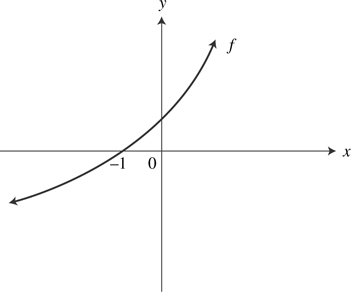The graph of f is shown above and f is twice differentiable. Which of the following has the smallest value?

I. f(–1)

II. f’(–1)

III. f"(–1)

10. A particle moves in the xy-plane so that its velocity vector at time t is v(t) = 〈 2 - 3t2, π sin (πt) 〉 and the particle’s position vector at time t = 2 is 〈4, 3〉 . What is the position vector of the particle when t = 3?